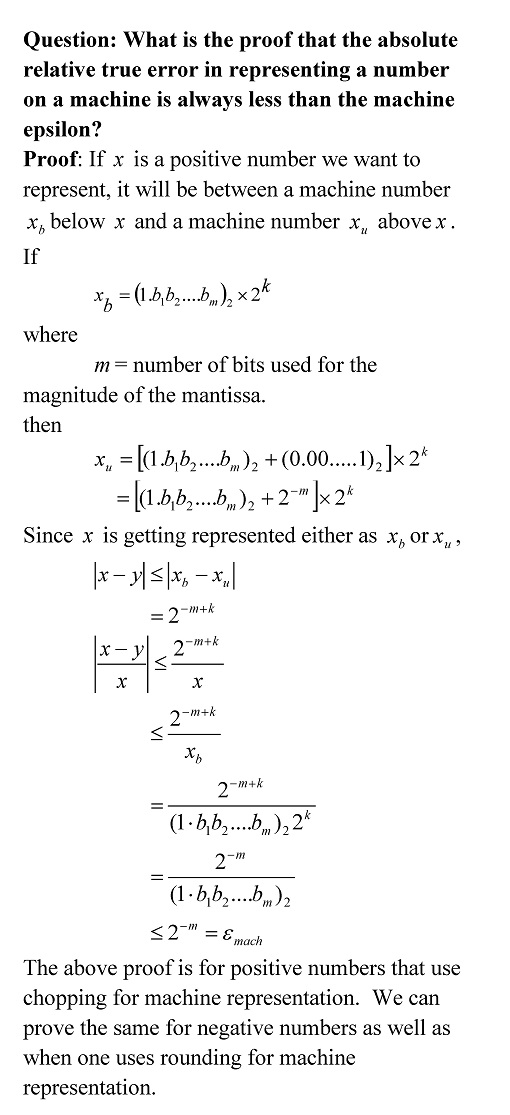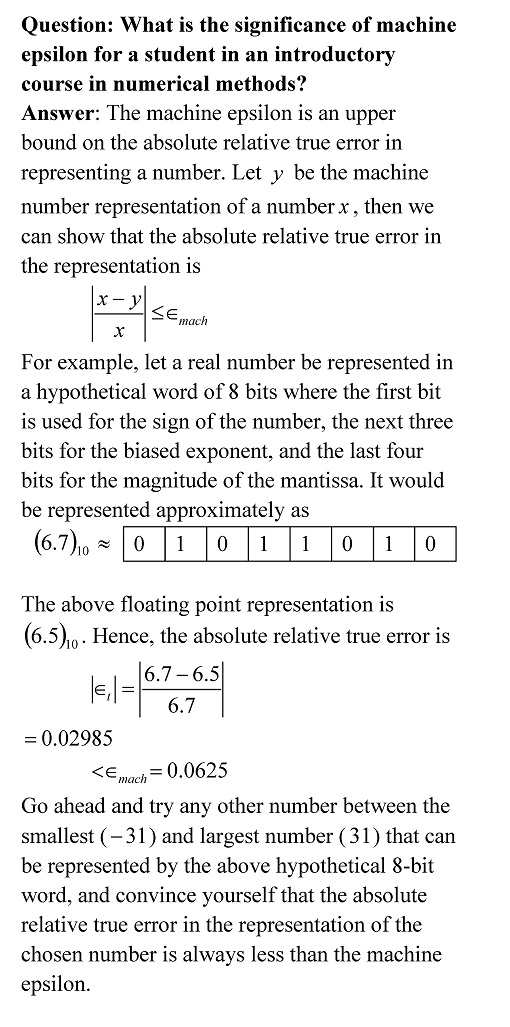## Machine epsilon – Question 5 of 5

In the previous blog posts, we answered

Here we answer the last question.• _________________

This post is brought to you by

• Holistic Numerical Methods Open Course Ware:
• the textbooks on
• the Massive Open Online Course (MOOCs) available at

## Repeated roots in ordinary differential equation – next independent solution – where does that come from?

When solving a fixed-constant linear ordinary differential equation where the characteristic equation has repeated roots, why do we get the next independent solution in the form of x^n*e^(m*x)?  Show this through an example.

See this pdf file for the answer.

________________________
This post is brought to you by

## Machine Epsilon – Question 4 of 5

In the previous blog posts, we answered

Here we answer the next question.Future post will answer this last question
Question 5 of 5: What is the proof that the absolute relative true error in representing a number on a machine is always less than the machine epsilon?

_________________

This post is brought to you by

• Holistic Numerical Methods Open Course Ware:
• the textbooks on
• the Massive Open Online Course (MOOCs) available at